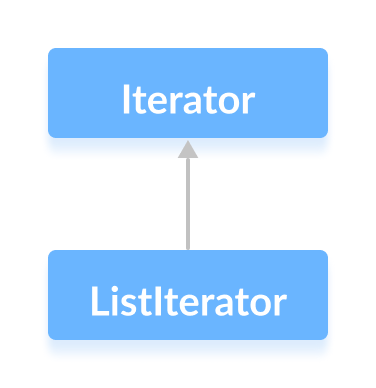# Java ListIterator Interface

The `ListIterator` interface of the Java collections framework provides the functionality to access elements of a list.

It is bidirectional. This means it allows us to iterate elements of a list in both the direction.

It extends the `Iterator` interface.The `List` interface provides a `listIterator()` method that returns an instance of the `ListIterator` interface.

## Methods of ListIterator

The `ListIterator` interface provides methods that can be used to perform various operations on the elements of a list.

• `hasNext()` - returns true if there exists an element in the list
• `next()` - returns the next element of the list
• `nextIndex()` returns the index of the element that the `next()` method will return
• `previous()` - returns the previous element of the list
• `previousIndex()` - returns the index of the element that the `previous()` method will return
• `remove()` - removes the element returned by either `next()` or `previous()`
• `set()` - replaces the element returned by either `next()` or `previous()` with the specified element

### Example 1: Implementation of ListIterator

In the example below, we have implemented the `next()`, `nextIndex()` and `hasNext()` methods of the `ListIterator` interface in an array list.

``````import java.util.ArrayList;
import java.util.ListIterator;

class Main {
public static void main(String[] args) {
// Creating an ArrayList
ArrayList<Integer> numbers = new ArrayList<>();
System.out.println("ArrayList: " + numbers);

// Creating an instance of ListIterator
ListIterator<Integer> iterate = numbers.listIterator();

// Using the next() method
int number1 = iterate.next();
System.out.println("Next Element: " + number1);

// Using the nextIndex()
int index1 = iterate.nextIndex();
System.out.println("Position of Next Element: " + index1);

// Using the hasNext() method
System.out.println("Is there any next element? " + iterate.hasNext());
}
}
``````

Output

```ArrayList: [1, 3, 2]
Next Element: 1
Position of Next Element: 1
Is there any next element? true
```

### Example 2: Implementation of ListIterator

In the example below, we have implemented the `previous()` and `previousIndex()` methods of the `ListIterator` interface in an array list.

``````import java.util.ArrayList;
import java.util.ListIterator;

class Main {
public static void main(String[] args) {
// Creating an ArrayList
ArrayList<Integer> numbers = new ArrayList<>();
System.out.println("ArrayList: " + numbers);

// Creating an instance of ListIterator
ListIterator<Integer> iterate = numbers.listIterator();
iterate.next();
iterate.next();

// Using the previous() method
int number1 = iterate.previous();
System.out.println("Previous Element: " + number1);

// Using the previousIndex()
int index1 = iterate.previousIndex();
System.out.println("Position of the Previous element: " + index1);
}
}
``````

Output

```ArrayList: [1, 3, 2]
Previous Element: 3
Position of the Previous Element: 0
```

In the above example, initially, the instance of the `Iterator` was before 1. Since there was no element before 1 so calling the `previous()` method will throw an exception.

We then used the `next()` methods 2 times. Now the `Iterator` instance will be between 3 and 2.

Hence, the `previous()` method returns 3.